Hamilton-Jacobi theory

A branch of classical variational calculus and analytical mechanics in which the task of finding extremals (or the task of integrating a Hamiltonian system of equations) is reduced to the integration of a first-order partial differential equation — the so-called Hamilton–Jacobi equation. The fundamentals of the Hamilton–Jacobi theory were developed by W. Hamilton in the 1820s for problems in wave optics and geometrical optics. In 1834 Hamilton extended his ideas to problems in dynamics, and C.G.J. Jacobi (1837) applied the method to the general problems of classical variational calculus.

The starting points of the Hamilton–Jacobi theory were established in the 17th century by P. Fermat and Chr. Huygens, who used the subject of geometrical optics for this purpose (cf. Fermat principle; Huygens principle). Below the footsteps of Hamilton are followed and the problem of propagation of light through an inhomogeneous (but, for the sake of simplicity, isotropic) medium, is considered whereis the local velocity of light at a point. According to Fermat's principle, light propagates from point to point in an inhomogeneous medium in shortest possible time. Let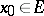be the starting point, and let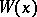be the shortest possible time for the light to traverse the distance fromto. The functionis known as the eikonal or the optical length of the path. It is assumed that during a short timethe light travels from the pointto the point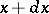. According to the Huygens principle, light will travel, apart from small magnitudes of a higher order, along the normal to the level surface of the function. Thus, the equationis satisfied, and the Hamilton–Jacobi equation for problems in geometrical optics follows:In analytical mechanics the role of Fermat's principle is played by the variational Hamilton–Ostrogradski principle, while the role of the eikonal is played by the action functional, i.e. by the integral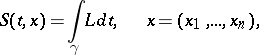(1)

along a trajectoryconnecting a given point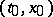with the point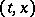, whereis the Lagrange function of the mechanical system.

It was suggested by Jacobi that a function resembling the action functional (1) should be used in solving all problems of classical variational calculus. The extremals of the problem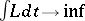issuing from the pointintersect the level surface of the principal function transversally (cf. Transversality condition); the form of the differential of the action functionalis deduced from this condition. Here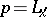, and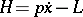is the Hamilton function (see also Legendre transform).

The last-mentioned relation yields the following equation for the function: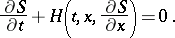(2)

This is the Hamilton–Jacobi equation.

The most important result of the Hamilton–Jacobi theory is Jacobi's theorem, which states that a complete integral of equation (2), i.e. the solution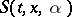of this equation, which will depend on the parameters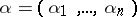(provided that), makes it possible to obtain the complete integral of the equation for the Euler functional (1) or, which is the same thing, of the Hamiltonian system connected with this functional, by the formulas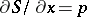,. The application of Jacobi's theorem to the integration of Hamiltonian systems is usually based on the method of separation of variables in special coordinates.

Despite the fact that the integration of partial differential equations is usually more difficult than solving ordinary equations, the Hamilton–Jacobi theory proved to be a powerful tool in the study of problems of optics, mechanics and geometry. The essence of Huygens' principle was used by R. Bellman in solving problems on optimal control.